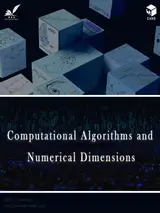# مجله الگوریتم های محاسباتی و ابعاد عددی

مجله الگوریتم های محاسباتی و ابعاد عددی

## Computational Algorithms and Numerical DimensionsComputational Algorithms and Numerical Dimensions (CAND) is an international scholarly , interdisciplinary, fully refereed, and quarterly free of charge journal as a non-commercial publication. The journal is intended to be accessible to a broad spectrum of researchers in numerical methods throughout science and engineering, and publishes original, review, case studies, and short communication contributions to all aspects of numerical and computational modeling including numerical analysis, numerical linear algebra, numerical engineering, differential/integral equations (ordinary, partial, fractional), scientific computing, computational mathematics, approximation and analytical methods, splitting and parallel computing, interpolation, orthogonal polynomials, computational complexity, variational inequalities, control, machine learning, and related areas of engineering and scientific applications.